# S6E3 - Calorimetry and Specific Heat Capacity | Sample Calculations

How exactly is "heat" measured experimentally?

## Calorimetry

Calorimetry  =  the science of measuring heat.

Calorimetry is based on observing the temperature change (ΔT) when a substance absorbs or gives off heat (q).

Some substances require a lot of heat energy (q) to raise their temperature by 1°C or 1K.

➞  these substances make good coolants (ex:  H2O).

Other substances don't require very much heat energy (q) to raise their temperature by 1°C or 1K.

Metals are a good example (ex:  Cu, Fe).

➞  a metal pan on a stove gets hotter much faster than the H2O in the pan!

==========

## Heat Capacity (C)

Heat Capacity  =  the amount of energy (as heat) required to raise the temperature of a substance by 1°C or 1K.

The higher the "heat capacity," the smaller the change in temperature for a given amount of absorbed heat.

➞  H2O has a high heat capacity (good coolant).

➞  Metals have low heat capacities.

----------

** The term "Heat Capacity" does not mention the amount of the substance being considered.

** If the heat capacity is given per gram of substance, it is called the "specific heat capacity."

----------

## Specific Heat Capacity (s)

Specific Heat Capacity  =  the energy (as heat) required to raise the temperature of 1g of a substance by 1°C or 1K.

Here are some common specific heat (s) values: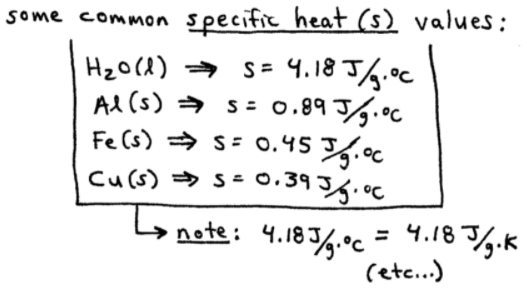==========

## The Calorimetry Equation

Actual measurements of heat gain and/or heat loss are performed using a device called a constant-pressure calorimeter.

Quite often, in your chemistry class or course, you'll hear the term "coffee-cup calorimeter."  This is just a type of constant-pressure calorimeter.

### Two Useful Calorimetry Equations:

1.  Heat Gained:  q  =  m . s . ΔT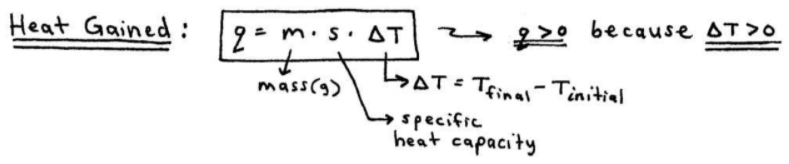2.  Heat Lost:  q  =  m . s . ΔT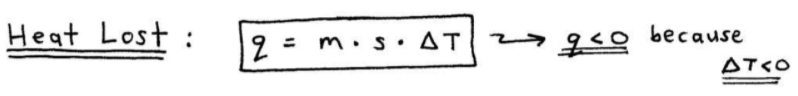----------

ex:  The specific heat capacity of silver is 0.24 J/g.°C

a.  Calculate the energy required to raise the temperature of 150.0 g of Ag from 273K to 298K.

q  =  m . s . ΔT  ,  where ΔT  =  Tfinal  -  Tinitial

q  =  (150.0g) . (0.24 J/g.°C) . (298K - 273K)

q  =  900. J

b.  Calculate the energy required to raise the temperature of 1.0 mol of Ag by 1°C.

... Well, we know the specific heat capacity (s) is 0.24 J/g.°C.

So we just need to convert the grams (g) part of the units to moles (mol):c.  It takes 1.25 kJ of energy to heat a sample of pure silver from 12.0°C to 15.2°C.  Calculate the mass of the silver sample.

(1.25 kJ / 1) . (1000 J / 1 kJ)  =  1250 J

q  =  m . s . ΔT

1250 J  =  (m) . (0.24 J/g.°C) . (15.2°C - 12.0°C)

1250 J  =  (m) . (0.24 J/g.°C) . (+ 3.2°C)

m = 1.6 x 103 g Ag

Here's the same math in an easy-to-follow, handwritten format: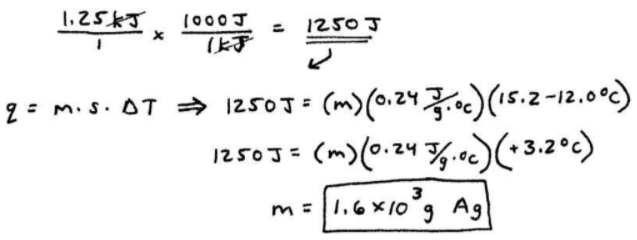==========

## Coffee-Cup Calorimetry Problems

Unlike the above example, where one substance is gaining or losing heat, often 2 substances at different initial temperatures are combined.

In situations like this, the heat lost by the hotter substance equals the heat gained by the cooler substance...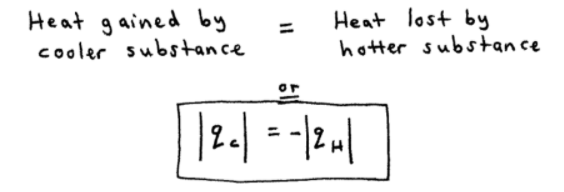We'll do some coffee-cup calorimeter practice problems in the next video...

==========

In my next video blog entry from SECTION 6 - Thermochemistry,

We'll start with some coffee-cup calorimetry examples, and then discuss the change in enthalpy, ΔH...